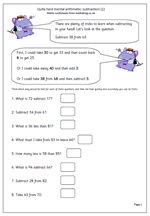# Subtracting mentally in Year 4Making the correct choice in deciding how to work out an answer is the key to fast mental arithmetic. Many children are unaware that there are often several ways of working out a calculation and that those who choose the best methods find maths easier and are able to answer questions more quickly and more accurately.

Let’s look at ‘Subtract 38 from 63.

This should be done, ‘in your head’ and will involve several stages, depending on the method used.

First, I could take 30 from 63 to leave 33. Then I could count back 8 from 33 which gives me 25.

Secondly, I could take 40 from 63 leaving 23 and then compensate by adding 2, which gives me 25.

Thirdly, I could take 38 from 68, leaving 30 and then compensate by subtracting 5 (because 63 is 5 less than 68).

There are several other ways very similar to these, but this does show that there is no ‘one right way, when it comes to mental arithmetic.

Quite hard mental arithmetic:_subtraction (1)